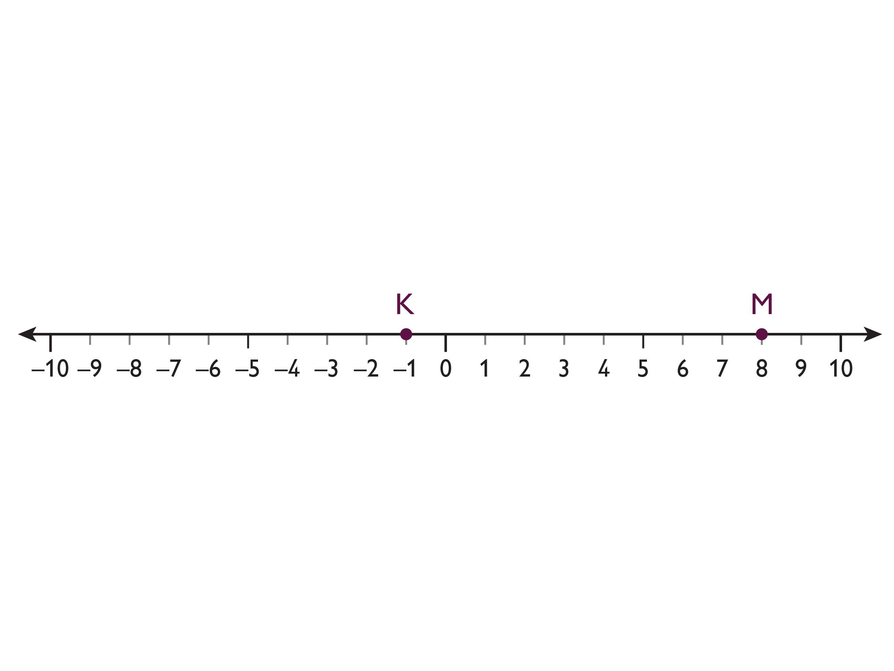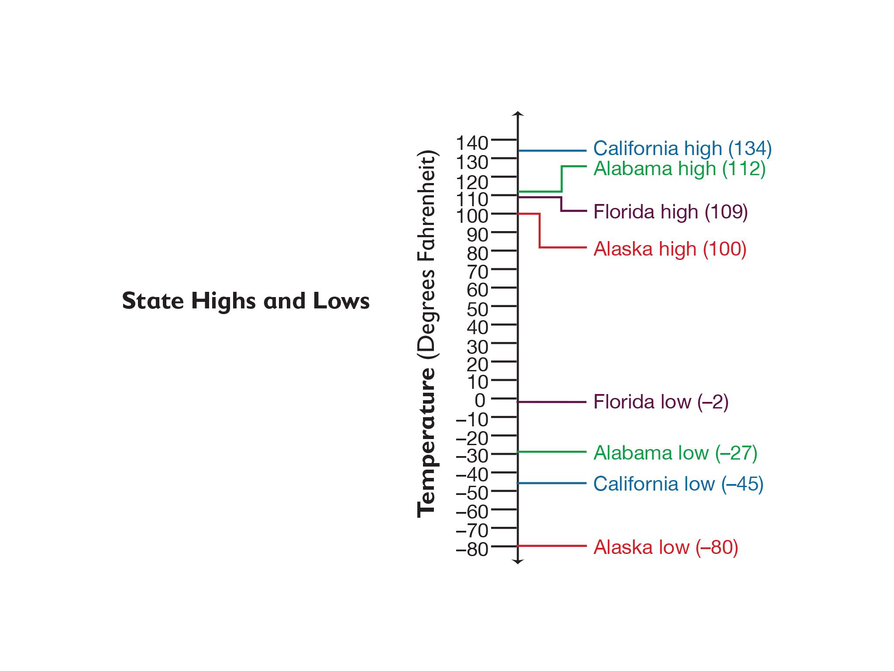Subject:
Numbers and Operations
Material Type:
Lesson Plan
Level:
Middle School
7
Provider:
Pearson
Tags:
Language:
English
Media Formats:
Text/HTML

# Understanding Distance & Coordinates## Overview

Students find the distance between points on a number line by counting and by using subtraction. They then use subtraction to find differences in temperatures.

Students discover that the distance between any two points on the number line is the absolute value of their difference, and apply this idea to solve problems.

# Key Concepts

Students know from earlier grades that the distance between two positive numbers on the number line can be found by subtracting the lesser number from the greater number. For example, the distance between 5 and 11 is 11 – 5, or 6. We can also state the rule for finding distance as “The distance between two positive numbers is the absolute value of their difference.” With this version of the rule, we don’t have to consider which number is greater; the result is the same either way. Using the example of 5 and 11, the distance is |11 – 5| or |5 – 11|, both of which are equal to 6.

This idea extends to the entire number line, including numbers to the left of 0. That is, the distance between any two numbers is the absolute value of their difference. For example, the distance between –5 and 3 is |–5 – 3| = |–8| = 8 or |3 – (–5)| = |8| = 8, and the distance between –12 and –7 is |–12 – (–7)| = |–5| = 5 or |–7 – (–12)| = |5| = 5.

# Goals and Learning Objectives

• Understand the relationship between the distance between two points on the number line and the difference in the coordinates of those points.
• Find distances in real-life situations.

# Lesson Guide

Discuss with students the number line in the Opening and what it represents. Be sure students see that the point labels are the first letters of the students’ names. Have students work in pairs and discuss the questions posed in the Opening.

Students should see that distance is the absolute value of the difference.

• Answers may vary. Possible answer: Yes, I agree; 8 − (−1) = 9, (−1) − 8  =−9. Another possible answer: No, I disagree; the distance is 9 blocks either way.
• Possible answer: To get from Karen’s house to Marcus’s house you have to walk 9 blocks east. We know this because to get from Karen’s house to Sophie’s house you have to walk 1 block east, and to go from Sophie’s house to Marcus’s house you have to walk 8 blocks east, for a total of 9 blocks east.
• Distance is always a positive number, so take the absolute value after subtracting.

# Distances on Long Street

Sophie and her friends Karen and Marcus all live on Long Street. This number line shows the locations of their houses. Each unit on the number line represents 1 block. Sophie’s house is located at 0. The house of the friend who lives to the west of Sophie is a negative number.

Karen’s house is at point K and Marcus’s house is at point M.

Karen says, “From my house to your house is 9 blocks.” She wrote the expression this way:

8 − (−1)

Marcus says, “Then from my house to your house, the distance is 9 blocks." He wrote the expression this way:

(−1) − 8

• Do you agree with Karen’s and Marcus’s statements? Explain why or why not.
• A distance is always a positive number. If you wanted to describe how to get from Marcus’s house to Karen’s house, what would you say?
• When subtraction is represented as distance, what would you need to do after you find the distance, in order to find the answer to the subtraction problem?# Math Mission

Discuss the Math Mission. Students will model integer subtraction by finding distances between points on a number line.

SWD: During partner work, monitor student discussions and provide guiding questions from the Interventions to help students see the mathematics and find their own way to a solution. If a student is struggling with a particular concept, hold an individual conference. If many students are struggling with a concept, pull a small group to discuss the concept.

## Opening

Model integer subtraction by finding distances between points on a number line.

# Lesson Guide

Have students work in pairs on the problems.

# Mathematics

The main focus of this lesson is subtraction as distance. When dealing with negative numbers, using the distance between two points helps students understand the subtraction. Student use a horizontal and a vertical number line to show the distance.

# Interventions

Student makes subtraction errors.

• Which two numbers are you subtracting? What is the subtraction problem?
• What is the rule for subtracting integers?
• How can you rewrite the subtraction problem as an addition problem?

• 17 − 3 = 14
• 3 − 15 = −12
• −7 − (−9) = 2
• 1 − (−5) = 6
• Answers will vary. Possible answer: Both number lines begin to the right of 0, move further right, and end at 7. The starting positions and the distance moved are different.

# Find Distances on a Number Line

• Use the Number Line Tool to model each subtraction problem as a distance on a number line. Write the difference as an equation.
• 17 − 3
• 3 − 15
• −7 − (−9)
• 1 − (−5)
• Create a problem in the form: positive number – negative number = 7

• Create another problem in the form: positive number – negative number = 7

• How do the number lines for the two problems you created compare? What is similar and what is different? Explain.

INTERACTIVE: Number Line Tool

## Hint:

A distance is always a positive number. So, how can you determine whether the answer to the subtraction problem is positive or negative?

# Lesson Guide

Have students continue  in pairs.

# Interventions

Student writes ab or ba (rather than |ab|) to calculate temperature differences.

• Remember that a temperature difference must be greater than or equal to 0. Will your expression always give a positive result?
• Test your expression for a = −6 and b = 2. Does it give a positive result?
• Test your expression for a = 3 and b = −4. Does it give a positive result?
• What can you do to make sure the value of your expression is always greater than or equal to 0, no matter what values a and b have?

• 134 − 109 = 25. The distance represents the difference between the two temperatures, 25 degrees. Since the number is positive, California’s high temperature is greater (higher) than Florida’s high temperature.
• 109 − (−2) = 111. Florida’s high temperature is 111 degrees higher than its low temperature.
• −80 − (−27) = −53. Alaska’s low temperature is 53 degrees lower than Alabama’s low temperature.
• 134 − (−80) = 214. California’s high temperature is 214 degrees higher than Alaska’s low temperature.

# Differences in Temperature

This number line shows very high and very low temperatures for different states in the United States.

• Find the distance between the point for the high temperature for California and the point for the high temperature for Florida. What does this distance represent?
• How much higher is the high temperature for Florida than the low temperature for Florida?
• How much lower is the low temperature for Alaska than the low temperature for Alabama?
• What is the difference in temperature between the high temperature for California and the low temperature for Alaska?## Hint:

The method you used to subtract on the horizontal number also works for a vertical number line.

# Preparing for Ways of Thinking

Look for students who show a variety of methods and use equations and number lines in different ways. Have these students present during Ways of Thinking.

ELL: In asking prompting questions, be sure to use an adequate pace with ELLs and be sure that they understand the meaning of the questions.

# Prepare a Presentation

Explain the method you used to subtract integers by looking at subtraction as the distance between two points. Give examples—both equations and number line representations—to support your explanation.

# Lesson Guide

Have students present their methods for subtracting integers.

ELL: When listening to pairs interact, be cognizant of the difficulties some ELLs encounter when they have to express themselves in a foreign language. Be sure to listen for meaning and not correctness of grammar. Encourage students to ask each other probing questions if the meaning is not apparent. This partner time will help students during the whole class discussion and the reflection activity.

# Ways of Thinking: Make Connections

Take notes about how your classmates used distance to subtract positive and negative numbers.

## Hint:

• Why can’t you just write ab to find the distance?

# Lesson Guide

Have students discuss the summary with a partner before turning to a whole class discussion. Use this opportunity to correct or clarify misconceptions.

SWD: Some students with disabilities may struggle to explain their mathematical reasoning in words. Provide additional sentence starters and/or paragraph frames to support students with this task.

# Summary of the Math: Subtraction as Distance

Subtraction can be looked at as the distance between two numbers.

You can use the number line to model the subtraction ab as distance, where a and b are any two numbers (positive or negative), as follows.

• Locate points a and b.
• Find the distance between points a and b. This value will be a positive number, because a distance is always positive.
• Determine the sign of the distance value in order to find the answer to the subtraction problem. The value of a − b is:
• Positive if a > b (if a is to the right of b on the number line).
• Negative if a < b (if a is to the left of b on the number line).

## Hint:

Can you:

• Explain the method for subtracting integers by finding the distance between two points on a number line?